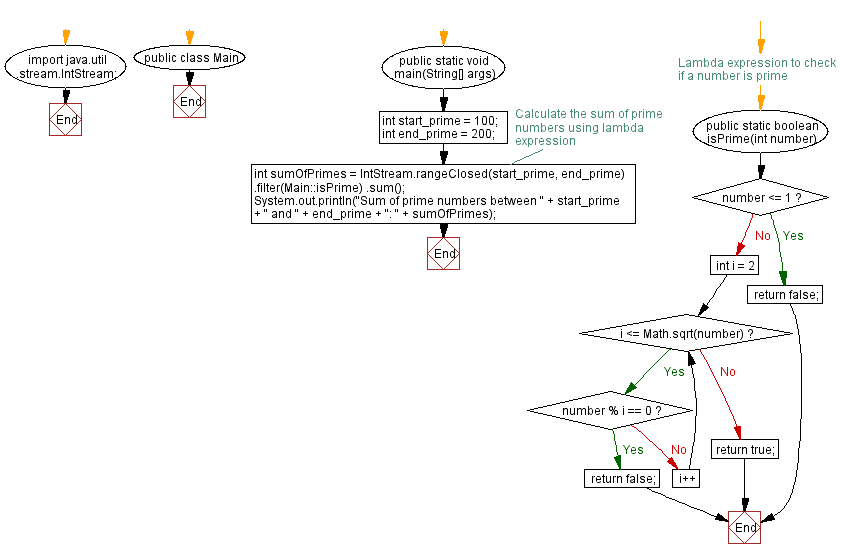# Java Program: Calculate sum of prime numbers with lambda expression

## Java Lambda Program: Exercise-21 with Solution

Write a Java program to implement a lambda expression to calculate the sum of all prime numbers in a given range.

Note: A prime number (or a prime) is a natural number greater than 1 that is not a product of two smaller natural numbers. A natural number greater than 1 that is not prime is called a composite number. For example, 5 is prime because the only ways of writing it as a product, 1 × 5 or 5 × 1, involve 5 itself. However, 4 is composite because it is a product (2 × 2) in which both numbers are smaller than 4. Primes are central in number theory because of the fundamental theorem of arithmetic: every natural number greater than 1 is either a prime itself or can be factorized as a product of primes that is unique up to their order.

Sample Solution:

Java Code:

``````import java.util.stream.IntStream;

public class Main {
public static void main(String[] args) {
int start_prime = 100;
int end_prime = 200;

// Calculate the sum of prime numbers using lambda expression
int sumOfPrimes = IntStream.rangeClosed(start_prime, end_prime)
.filter(Main::isPrime)
.sum();
System.out.println("Sum of prime numbers between " + start_prime + " and " + end_prime + ": " + sumOfPrimes);
}
// Lambda expression to check if a number is prime
public static boolean isPrime(int number) {
if (number <= 1) {
return false;
}
for (int i = 2; i <= Math.sqrt(number); i++) {
if (number % i == 0) {
return false;
}
}
return true;
}
}
``````

Sample Output:

```Sum of prime numbers between 100 and 200: 3167
```

Explanation:

In the above exercise,

• The start_prime and end_primevariables define the range of numbers within which we want to calculate the sum of prime numbers.
• The lambda expression Main::isPrime filters out non-prime numbers from the range of numbers.
• The isPrime method is a lambda expression itself, which checks whether a given number is prime or not using a simple algorithm.b.

Flowchart:Live Demo:

Java Code Editor:

Improve this sample solution and post your code through Disqus

Java Lambda Exercises Previous: Sort list of objects with lambda expression.
Java Lambda Exercises Next: Lambda expression for checking uppercase, lowercase, or mixedcase strings.

What is the difficulty level of this exercise?

Test your Programming skills with w3resource's quiz.

﻿

## Java: Tips of the Day

How do I remove repeated elements from ArrayList?

If you don't want duplicates in a Collection, you should consider why you're using a Collection that allows duplicates. The easiest way to remove repeated elements is to add the contents to a Set (which will not allow duplicates) and then add the Set back to the ArrayList:

```Set<String> set = new HashSet<>(yourList);
yourList.clear();